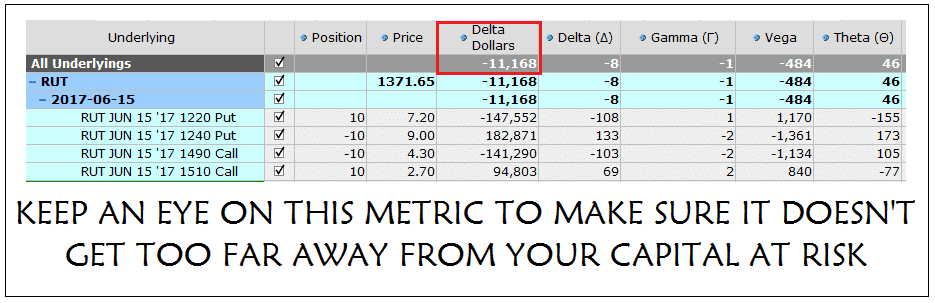Delta is one of the four main option greeks, and any serious trader needs to have a thorough understanding of this greek if they hope to have any chance of success in the trading options.

Most serious traders probably have a pretty good grasp of delta.

However, there is one metric related to delta that I use religiously in my trading and that is Delta Dollars.

WHAT IS DELTA DOLLARS?

Delta dollars is quite simply the position delta x the underlying price.

We know that delta gives us the share equivalency ratio, so if we own a long call with delta 0.40 it is equivalent to being long 40 shares of the underlying.

Let’s assume the stock is trading at \$100. The delta dollars figure would be 40 x \$100 = \$4,000.

This tells us that the option position is equivalent to having \$4,000 invested in the stock.

The delta dollars figure is going to depend a lot on the price of the stock. Let’s say that instead of the stock trading at \$100, it was trading at \$500. Our delta dollars figure in this example would be 40 x \$500 = \$20,000.

Perhaps now you can understand why it’s important to look at the delta dollars number and not just the delta.

WHY IS IT IMPORTANT

Delta dollars tells us our overall directional exposure in the market.

If our account size is \$50,000 and out delta is 100, that doesn’t really tell us much.

But if our delta dollars exposure is \$200,000 then we know that it is too high for our account size.

Personally, I like to set a rule that I don’t let my delta dollar exposure get above 150% of my account size. More conservative traders might like to set that rule at 100%, whereas more aggressive traders might set it at 200%.

It’s personal preference, but the first step as a delta neutral trader is to start paying attention to delta dollars and then develop rules around this metric.

I also have rules regarding the delta dollar exposure for each trade and strategy.

PRACTICAL EXAMPLE

For an iron condor, I usually set a 200% rule for Delta Dollars.

Assume you have an iron condor on RUT that is risking \$20,000. If the Delta Dollars figure gets above plus or minus \$40,000 you might want to think about adjusting and getting back closer to neutral.